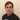# Leetcode 234 Palindrome Linked List

February 04, 2021

Welcome back, part of my Self Development Goals for 2021 is “Complete at least 25 - 50 Leetcode Questions”, today we are going to discuss and solve Palindrome Linked List

## Problem

“A palindrome is a sequence that is the same if it is reversed e.g a palindrome for 121 is 121, or abc -> cba”

## Extra space O(n) Solution

We can solve this by go through the entire linked list and add each value to the array, once we have each value in the array we can then use two pointers, one at start of array and another at end of array, as long as the strings are equal we know its still a palindrome.

``````# Definition for singly-linked list.
# class ListNode:
#     def __init__(self, val=0, next=None):
#         self.val = val
#         self.next = next
class Solution:
def isPalindrome(self, head: ListNode) -> bool:
"""
:rtype: bool

Go through array using two pointers, if at any point they are not the same return false, if we make it to end of list return True

Complexity: O(n + m) = O(n)
Space: O(n) - Array
"""

arr = []

# Check if its palindrome by using two pointers (one at start of array and one at end, comparing them as we go)
l = 0
r = len(arr) - 1;

while l < r:
if arr[l] != arr[r]:
return False
l = l + 1;
r = r -1;
return True``````

### Time / Space Complexity

Time: O(N + N) = O(N)

Why: We need go through each element of the linked list and add it to an array then go through each element of the new array again to check if its a palindrome so its O(n + n) which is O(n)

Space: O(N)

Why: We are storing each value in an array so the space is O(N)

## Constant Space O(1) Solution

The constant space solution is a bit more difficult to understand, “pointers” can be hard to get your head around at first but the more you use them the easier it becomes. To check if a Linked list is a palindrome in place it requires a bit of thinking…lets start with how would we check if an array or a string is a palindrome, from the solution above we know we would have a pointer at the start and at end of the string / list and moving the pointers backwards and forward checking if they are equal. Can we do the same thing in a linked list? Well the main problem with a singly-linked list is that we don’t have a way to move the pointer “back” (unlike an array) to check if each value is equal (at least without a doubly linked list) so what can we do?

What if we could somehow reverse only the second half of the list? Imagine you have a list like: 1 -> 2 -> 3 -> 2 -> 1 and we could somehow reverse the 2nd half to look like: 1st half: 1 -> 2 -> 3 2nd half: 1 -> 2 -> 3 (now reversed)

Then we could have two pointers at the start of each half and compare them! If you have been following this series of blog posts so far this solution will require us to revisit back two of them:

The idea is, we find the middle of the linked list, we take the pointer pointing at the middle of the linked list and reverse everything from there. Once we have the reversed 2nd half we can traverse the half’s with two pointers and compare at each point. If at any point they are not equal we can say it is not a palindrome.

``````# Definition for singly-linked list.
# class ListNode:
#     def __init__(self, val=0, next=None):
#         self.val = val
#         self.next = next
class Solution:
def isPalindrome(self, head: ListNode) -> bool:

return True

# Find middle of list
while fast and fast.next:
slow = slow.next
fast = fast.next.next

# Reverse from slow to end
slow = self.reverse(slow)

# Since we have reversed the 2nd part of the linked list, we can now go through each node and compare slow and fast values, fast will point to start -> middle, slow will point from middle to end (reversed)

while slow != None:
if slow.val != fast.val:
return False

slow = slow.next
fast = fast.next

return True

def reverse(self, currentNode: ListNode):
prev = None
while currentNode != None:
nextNode = currentNode.next
currentNode.next = prev
prev = currentNode
currentNode = nextNode

return prev``````

### Time / Space Complexity

Time: O(N)

Why: We need go through each element of the linked list once until we fast pointer reaches end and slow is around the middle of the list. We then reverse the 2nd half of the list and finally go through the list again to check if they values are equal.

Space: O(1)

Why: Since we are switching pointers in place we are using constant space, we never create an extra space so its O(1)

## Conclusion

I hope you enjoyed this second post on solving some Leetcode problems, Anyway, that is 9 / 25 for my yearly goal done! now onto the rest, i hope you enjoyed this post!

Until next time

JasonPersonal Blog by Jason Lloyd.
I talk about programming, life, self-development and everything in-between.